# KS1 Maths Quiz - Year 2 Position, Direction and Movement (Questions)

This quiz addresses the requirements of the National Curriculum KS1 Maths and Numeracy for children aged 6 and 7 in year 2. Specifically this quiz is aimed at the section dealing with using mathematical vocabulary to describe position, direction and movement, including movement in a straight line and distinguishing between rotation as a turn and in terms of right angles for quarter, half and three-quarter turns (clockwise and anti-clockwise).

Knowing and using the languages of position, direction and movement is a skill developed in Year 2. A good understanding of words such as left and right, top, middle and bottom, on top of, in front of, above, between, around, near, close and far, up and down, forwards and backwards, inside and outside, which describe position and movement, will help Year 2 children move on to talk about rotating and turning in both directions.

To see a larger image, click on the picture.
1. This child is running...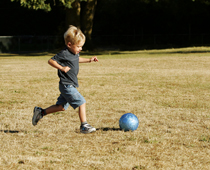[ ] forwards [ ] sideways [ ] backwards [ ] underneath
2. At the moment, the cucumber slices are near the bottom of the picture.
If I gave the plate half a turn, where would they be?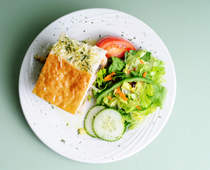[ ] At the bottom of the picture [ ] On the left of the picture [ ] On the right of the picture [ ] At the top of the picture
3. If I turned this picture a quarter turn anticlockwise, which way would the stick be pointing?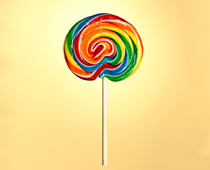[ ] To the left [ ] Straight up [ ] Straight down [ ] To the right
4. If the needle turned a quarter of a turn clockwise, which word would the red tip point to?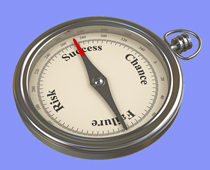[ ] Success [ ] Chance [ ] Failure [ ] Risk
5. If the minute hand makes a three-quarter turn clockwise, which number will it be pointing to?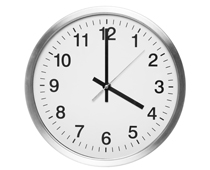[ ] 12 [ ] 6 [ ] 5 [ ] 9
6. If she blows and the windmill makes 3 full turns, what colour would be at the top?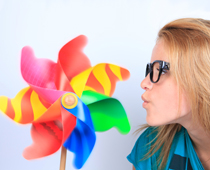[ ] Pink [ ] Green [ ] Blue [ ] Red
7. These arrows tell us that traffic should move around the roundabout in which direction?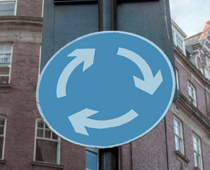[ ] Anticlockwise [ ] Clockwise [ ] Forwards [ ] Backwards
8. If they walk forwards, what is most likely to happen first?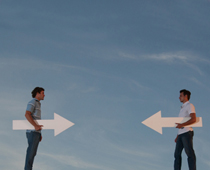[ ] They will jump in the air [ ] The tips of the arrows will meet [ ] They will fall off [ ] They will start to run
9. If he gave his arrow half a turn, in which direction would it point?[ ] Straight down [ ] Straight up [ ] To the right [ ] To the left
10. In order to turn the hob up to 3, she should...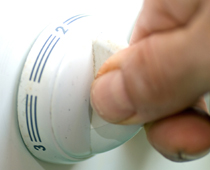[ ] Pull hard [ ] Turn the knob in an anticlockwise direction [ ] Turn the knob in a clockwise direction [ ] Press hardKS1 Maths Quiz - Year 2 Position, Direction and Movement (Answers)
1. This child is running...[x] forwards [ ] sideways [ ] backwards [ ] underneath
He is chasing the ball and running in a straight line
2. At the moment, the cucumber slices are near the bottom of the picture.
If I gave the plate half a turn, where would they be?[ ] At the bottom of the picture [ ] On the left of the picture [ ] On the right of the picture [x] At the top of the picture
Turning clockwise or anticlockwise would give the same result
3. If I turned this picture a quarter turn anticlockwise, which way would the stick be pointing?[ ] To the left [ ] Straight up [ ] Straight down [x] To the right
Turning anticlockwise means turning the opposite way the clock hands travel
4. If the needle turned a quarter of a turn clockwise, which word would the red tip point to?[ ] Success [x] Chance [ ] Failure [ ] Risk
The arrow would turn the same way the clock hands travel
5. If the minute hand makes a three-quarter turn clockwise, which number will it be pointing to?[ ] 12 [ ] 6 [ ] 5 [x] 9
Three quarters is the same as 45 minutes on the clock face
6. If she blows and the windmill makes 3 full turns, what colour would be at the top?[ ] Pink [ ] Green [ ] Blue [x] Red
Every full turn would result in everything being back at the starting position
7. These arrows tell us that traffic should move around the roundabout in which direction?[ ] Anticlockwise [x] Clockwise [ ] Forwards [ ] Backwards
Everyone travelling in this way means fewer accidents!
8. If they walk forwards, what is most likely to happen first?[ ] They will jump in the air [x] The tips of the arrows will meet [ ] They will fall off [ ] They will start to run
They are facing each other, so walking forwards means they will meet
9. If he gave his arrow half a turn, in which direction would it point?[ ] Straight down [ ] Straight up [ ] To the right [x] To the left
Half a turn means pointing in the opposite direction
10. In order to turn the hob up to 3, she should...[ ] Pull hard [ ] Turn the knob in an anticlockwise direction [x] Turn the knob in a clockwise direction [ ] Press hard
To get from 2 to 3, the knob would have to turn to the right